# Combinatorial calculator

Find out how many different ways you can choose k items from n items set. With/without repetition, with/without order.

(n)
(k)

# A bit of theory - foundation of combinatorics

## Variations

A variation of the k-th class of n elements is an ordered k-element group formed from a set of n elements. The elements are not repeated and depend on the order of the group's elements (therefore arranged).

The number of variations can be easily calculated using the combinatorial rule of product. For example, if we have the set n = 5 numbers 1,2,3,4,5 and we have to make third-class variations, their V3 (5) = 5 * 4 * 3 = 60.
${V}_{k}\left(n\right)=n\left(n-1\right)\left(n-2\right)\mathrm{.}\mathrm{.}\mathrm{.}\left(n-k+1\right)=\frac{n!}{\left(n-k\right)!}$
n! we call the factorial of the number n, which is the product of the first n natural numbers. The notation with the factorial is only clearer, equivalent. For calculations, it is fully sufficient to use the procedure resulting from the combinatorial rule of product.

## Permutations

The permutation is a synonymous name for a variation of the nth class of n-elements. It is thus any n-element ordered group formed of n-elements. The elements are not repeated and depend on the order of the elements in the group.
$P\left(n\right)=n\left(n-1\right)\left(n-2\right)\mathrm{.}\mathrm{.}\mathrm{.}1=n!$
A typical example is: We have 4 books, and in how many ways can we arrange them side by side on a shelf?

## Variations with repetition

A variation of the k-th class of n elements is an ordered k-element group formed of a set of n elements, wherein the elements can be repeated and depends on their order. A typical example is the formation of numbers from the numbers 2,3,4,5, and finding their number. We calculate their number according to the combinatorial rule of the product:
${V}_{k}^{\mathrm{\prime }}\left(n\right)=n\cdot n\cdot n\cdot n\mathrm{.}\mathrm{.}\mathrm{.}n={n}^{k}$

## Permutations with repeat

A repeating permutation is an arranged k-element group of n-elements, with some elements repeating in a group. Repeating some (or all in a group) reduces the number of such repeating permutations.
${P}_{{k}_{1}{k}_{2}{k}_{3}\mathrm{.}\mathrm{.}\mathrm{.}{k}_{m}}^{\mathrm{\prime }}\left(n\right)=\frac{n!}{{k}_{1}!{k}_{2}!{k}_{3}!\mathrm{.}\mathrm{.}\mathrm{.}{k}_{m}!}$
A typical example is to find out how many seven-digit numbers formed from the numbers 2,2,2, 6,6,6,6.

## Combinations

A combination of a k-th class of n elements is an unordered k-element group formed from a set of n elements. The elements are not repeated, and it does not matter the order of the group's elements. In mathematics, disordered groups are called sets and subsets. Their number is a combination number and is calculated as follows:
${C}_{k}\left(n\right)=\left(\genfrac{}{}{0px}{}{n}{k}\right)=\frac{n!}{k!\left(n-k\right)!}$
A typical example of combinations is that we have 15 students and we have to choose three. How many will there be?

## Combinations with repeat

Here we select k element groups from n elements, regardless of the order, and the elements can be repeated. k is logically greater than n (otherwise, we would get ordinary combinations). Their count is:
${C}_{k}^{\mathrm{\prime }}\left(n\right)=\left(\genfrac{}{}{0px}{}{n+k-1}{k}\right)=\frac{\left(n+k-1\right)!}{k!\left(n-1\right)!}$
Explanation of the formula - the number of combinations with repetition is equal to the number of locations of n − 1 separators on n-1 + k places. A typical example is: we go to the store to buy 6 chocolates. They offer only 3 species. How many options do we have? k = 6, n = 3.

## Foundation of combinatorics in word problems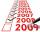Determine the number of integers from 1 to 106 with ending four digits 2015.
• Scrap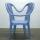From 6 products are 3 scrap. What is the probability that the random pick of 2 products have no defective product?
• Probability - tickets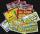What is the probability when you have 25 tickets in 5000 that you not wins the first (one) prize?
• DicesWe will throw two dice. What is the probability that the ratio between numbers on first and second dice will be 1:2?
• Chess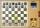How many different ways can initiate a game of chess (first pass)?
• DivisionThe division has 18 members: 10 girls and six boys, two leaders. How many different patrols can be created if one patrol is two boys, three girls, and one leader?
• PIN - codesHow many five-digit PIN - code can we create using the even numbers?
• Win in raffle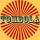The raffle tickets were sold 200, 5 of which were winning. What is the probability that Peter, who bought one ticket will win?
• One dice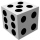Calculate the probability of one dice roll with the numbers 1, 2, 3, 4, 5, 6 on the walls. Write the results in a notebook in the shape of a fraction in the basic form: 2/3. a, The number 1 falls on the cube. b, The number 5 falls on the cube. c, An even
• Smoker male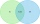For a person selected randomly from a certain population, events A and B are defined as follows. A = event the person is male B = event the person is a smoker. For this particular population, it is found that P(A ) = 0.53, P(B) = 0.15, and P(A n B ) = 0.1
• Commitee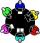A class consists of 6 males and 7 females. How many committees of 7 are possible if the committee must consist of 2 males and 5 females?
• Football league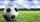In the football league is 16 teams. How many different sequence of results may occur at the end of the competition?
• Raffle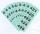In the pool is numbers from 1 to 115. What is the probability that a randomly selected number is not a prime number?
• Orchard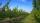10 trees in 5 lines grows in the orchard. How many trees are in the orchard?
• Points in planeThe plane is given 12 points, 5 of which are located on a straight line. How many different lines could be drawn from these points?
• Alarm systems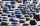What is the probability that at least one alarm system will signal the theft of a motor vehicle when the efficiency of the first system is 90% and of the independent second system 80%?